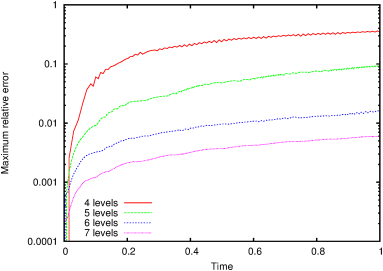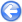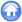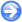### 13.2PASS: Advection of a cosine bell around the sphere

Author
Stéphane Popinet
Command
sh cosine.sh
Version
091029
Required files
cosine.sh isolines.gfv reference.gfv zero.gfv error-45.ref error-90.ref rossmanith45 rossmanith90
Running time
2 minutes 44 seconds

This test case was suggested by Williamson et al.  (Problem #1). A "cosine bell" initial concentration is given by

 h(λ,θ)=(h0/2)(1+cos(π r/R))

if r<R and 0 otherwise, with R=1/3 and

 r=arccos[sinθcsinθ+cosθccosθcos(λ−λc)]

the great circle distance between longitude, latitude (λ,θ) and the center initially taken as (λcc)=(3π/2,0).

The advection velocity field corresponds to solid-body rotation at an angle α to the polar axis of the spherical coordinate system. It is given by the streamfunction

 ψ=−u0(sinθcosα−cosλcosθsinα)

The cosine bell field is rotated once around the sphere and should come back exactly to its original position. The difference between the initial and final fields is a measure of the accuracy of the advection scheme coupled with the spherical coordinate mapping (the "conformal expanded spherical cube" metric in our case).

For the "spherical cube" metric, two angles are considered: 45 degrees which rotates the cosine bell above four of the eight "poles" of the mapping and 90 degrees which avoids the poles entirely. Mass is conserved to within machine accuracy in either case.

The mesh is adapted dynamically according to the gradient of tracer concentration.

 Figure 161: Tracer field after one rotation around the sphere with α=45∘ (red). Reference solution (green). Zero level contour line (blue). Equivalent static resolutions (a) 16× 16× 6. (b) 32× 32× 6. (c) 64× 64× 6. (d) 128× 128× 6.
 (a)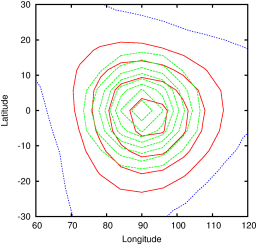(b)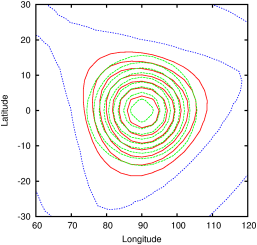(c)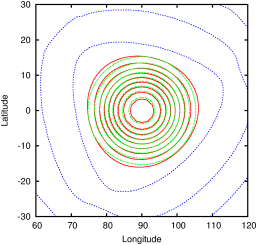(d)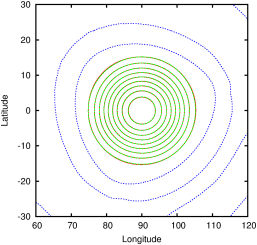Figure 162: Tracer field after one rotation around the sphere with α=90∘ (red). Reference solution (green). Zero level contour line (blue). Equivalent static resolutions (a) 16× 16× 6. (b) 32× 32× 6. (c) 64× 64× 6. (d) 128× 128× 6.
 (a)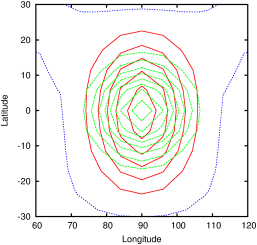(b)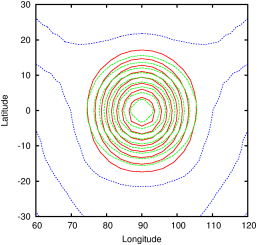(c)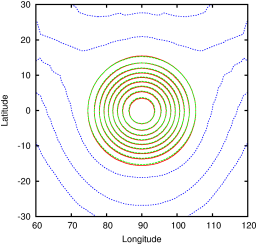(d)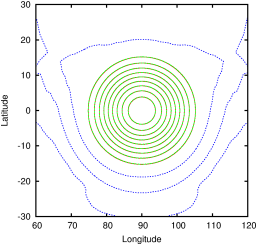Figure 163: Relative error norms (as defined in ) as functions of spatial resolution. The results of Rossmanith  using a gnomonic spherical cube metric and a different 2nd-order advection scheme are also reproduced for comparison. (a) α=45∘. (b) α=90∘.
 (a)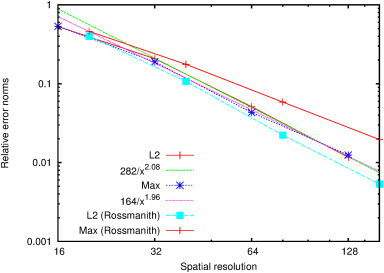(b)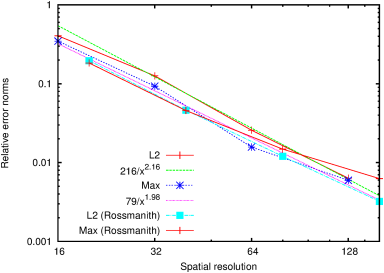Figure 164: Maximum relative errors as functions of time. (a) α=45∘. (b) α=90∘.
 (a)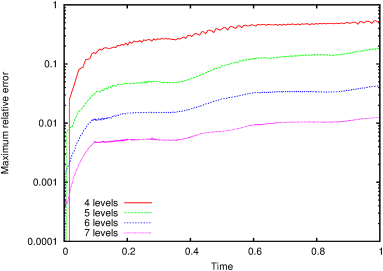(b)